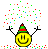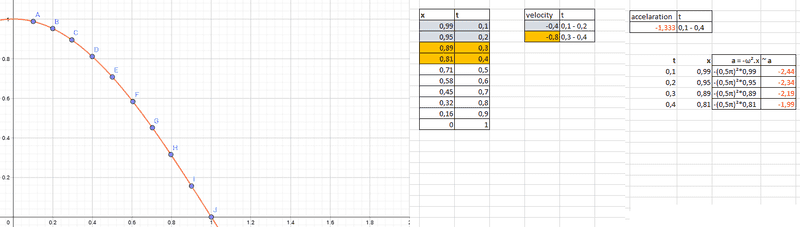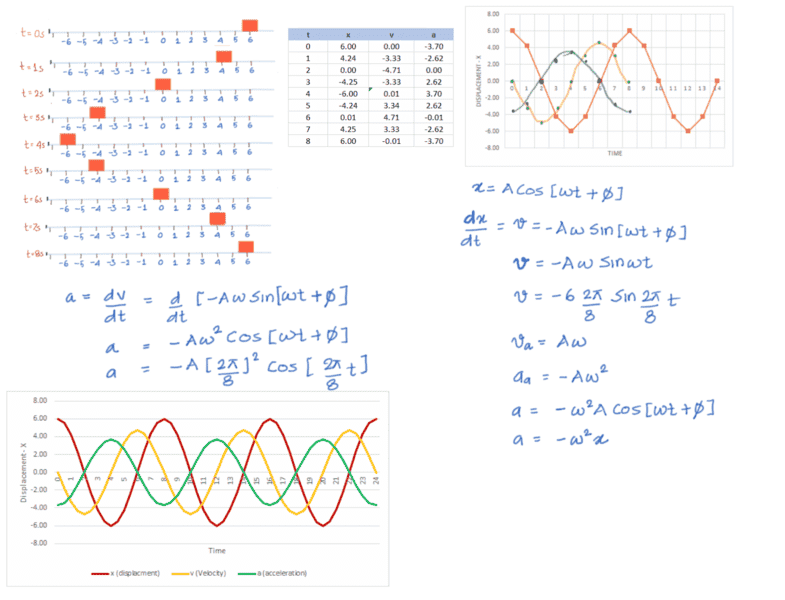# Acceleration in SHM

Hello guys!

Today I was studying SHM and I can't understand how to proof that a = -ω2Acos(ωt+φ) gives me accelaration of particle executing simple harmonic motion!

If someone "build" this equation step-by-step I would be really thankfull!

:)

kuruman
Homework Helper
Gold Member
2021 Award
Hi Vinicius and welcome to PF.

In your own words, what does SHM mean to you and how would you recognize it if you saw it?

jtbell
Mentor
I can't understand how to proof that a = -ω2Acos(ωt+φ) gives me accelaration of particle executing simple harmonic motion!

Have you studied calculus? (specifically, derivatives)

Last edited:
•Chestermiller
Hi Vinicius and welcome to PF.

In your own words, what does SHM mean to you and how would you recognize it if you saw it?

Hello Kuroman! Thank you!When I think in SHM I instantly remember a pendulum or a a block connect in a spring. I imagine SHM a classification of this kind of moviment that "always come back and repeats." Oh, and I also remember about trigonometric functions and some sin or cos graphics.

For example, I understand how to get the equation v = Aω cos (ωt + φ) and how/where it works. But I have some difficult in "see" that accelaration part.

Have you studied calculus? (specifically, derivatives)

I know the main concepts (e.g. how to calculate the velocity of a particle in a certain point, how to find the position of a particle in a SHM, I understand those equations x = A cos ( ωt) v = - Aω sin ( ωt)) but I don't understand that accelaration part!

For example, I saw this explanation:

"At the extreme ends, when a spring is at its maximum stretch or compress, the spring force is at its maximum magnitude, and therefore the acceleration it gives to the attached mass is maximum. We may therefore state that: " In Simple Harmonic Motion, the maximum of acceleration magnitude occurs at x = +/-A (the extreme ends where force is maximum), and acceleration at the middle ( at x = 0 ) is zero.

Using Calculus, if the equation for x is

x = A cos ( ωt), then v, and a are derived as follows:

v = (dx /dt) = - Aω sin ( ωt) ; and

a = (d2x /dt2) = -Aω2 cos ( ωt)."
http://www.pstcc.edu/departments/natural_behavioral_sciences/Web Physics/Chapter015.htm

Unfortunately I did not study calculus yet. Is there another way to understand this part? Do you recommend that I study calculus and understand this later?

kuruman
Homework Helper
Gold Member
2021 Award
Although calculus is certainly helpful, it is not necessary to understand "Oscillatory Simple Harmonic Motion" (OSHM). The "oscillatory" part means that the motion repeats itself after a certain time interval. Not all repeating motion is simple harmonic, a beating heart for example. The "harmonic" part means sines and cosines are used to model the description of the motion. The "simple" part means that single frequency sines and cosines are needed, not a superposition of many frequencies.
The hallmark of OSHM is that the acceleration is proportional to the negative of the displacement, the constant of proportionality being the square of the frequency ##\omega##. That is ##a=-\omega^2x##. Here, ##x## is the displacement from the equilibrium position, i.e. where the net external force (or torque) is zero. Can you answer the question now?

Although calculus is certainly helpful, it is not necessary to understand "Oscillatory Simple Harmonic Motion" (OSHM). The "oscillatory" part means that the motion repeats itself after a certain time interval. Not all repeating motion is simple harmonic, a beating heart for example. The "harmonic" part means sines and cosines are used to model the description of the motion. The "simple" part means that single frequency sines and cosines are needed, not a superposition of many frequencies.
The hallmark of OSHM is that the acceleration is proportional to the negative of the displacement, the constant of proportionality being the square of the frequency ##\omega##. That is ##a=-\omega^2x##. Here, ##x## is the displacement from the equilibrium position, i.e. where the net external force (or torque) is zero. Can you answer the question now?

Hmm... It makes sense now. I mean, if x = 0 (equilibrium position) in a SHM the acceleration should be zero (there's no force acting). And in extremes it gets the highest amount of acceleration.
I understand it now!Thanks, really! thank you!

##a=-\omega^2x##.
##a=-\omega^2Acos(\omega t + \phi)##

CWatters
Homework Helper
Gold Member
Got some graph paper?.. Try plotting a graph of position Vs time for one cycle on a large scale (eg fill the page). Then...

Presumably you know that the velocity at any time is the rate of change in position, or the slope of the position graph? So measure the slope on the position graph at various points carefully and plot a graph of velocity Vs time on another sheet.

Likewise acceleration is the rate of change of velocity, or the slope of the velocity graph. You could repeat the process and plot a graph of acceleration Vs time but by now you should have spotted the pattern so this may not be necessary.

Got some graph paper?.. Try plotting a graph of position Vs time for one cycle on a large scale (eg fill the page). Then...

Presumably you know that the velocity at any time is the rate of change in position, or the slope of the position graph? So measure the slope on the position graph at various points carefully and plot a graph of velocity Vs time on another sheet.

Likewise acceleration is the rate of change of velocity, or the slope of the velocity graph. You could repeat the process and plot a graph of acceleration Vs time but by now you should have spotted the pattern so this may not be necessary.

I did it:Later I will try to get accelaration with more specifics values.
Thanks for the tip!#### Attachments

•test.png
63.3 KB · Views: 635
CWatters
Homework Helper
Gold Member
You should approach answer to this question quite like you would do for motion in 1,2 or 3 dimension.
In the picture below start from the derivation on the RHS where position x of the particle is given as a function of t (x = A cost (wt + phi)
Take the first derivative of X w.r.t. and you get velocity.
Take second derivative of X w.r.t and you get acceleration.

You can actually plot all x,v and a values with time using these equations (see the table below) and you can plot them on a graph and you will get sin curves for x,v and a (and yes remember that even though a is a cos function, you can still call it a sine wave)